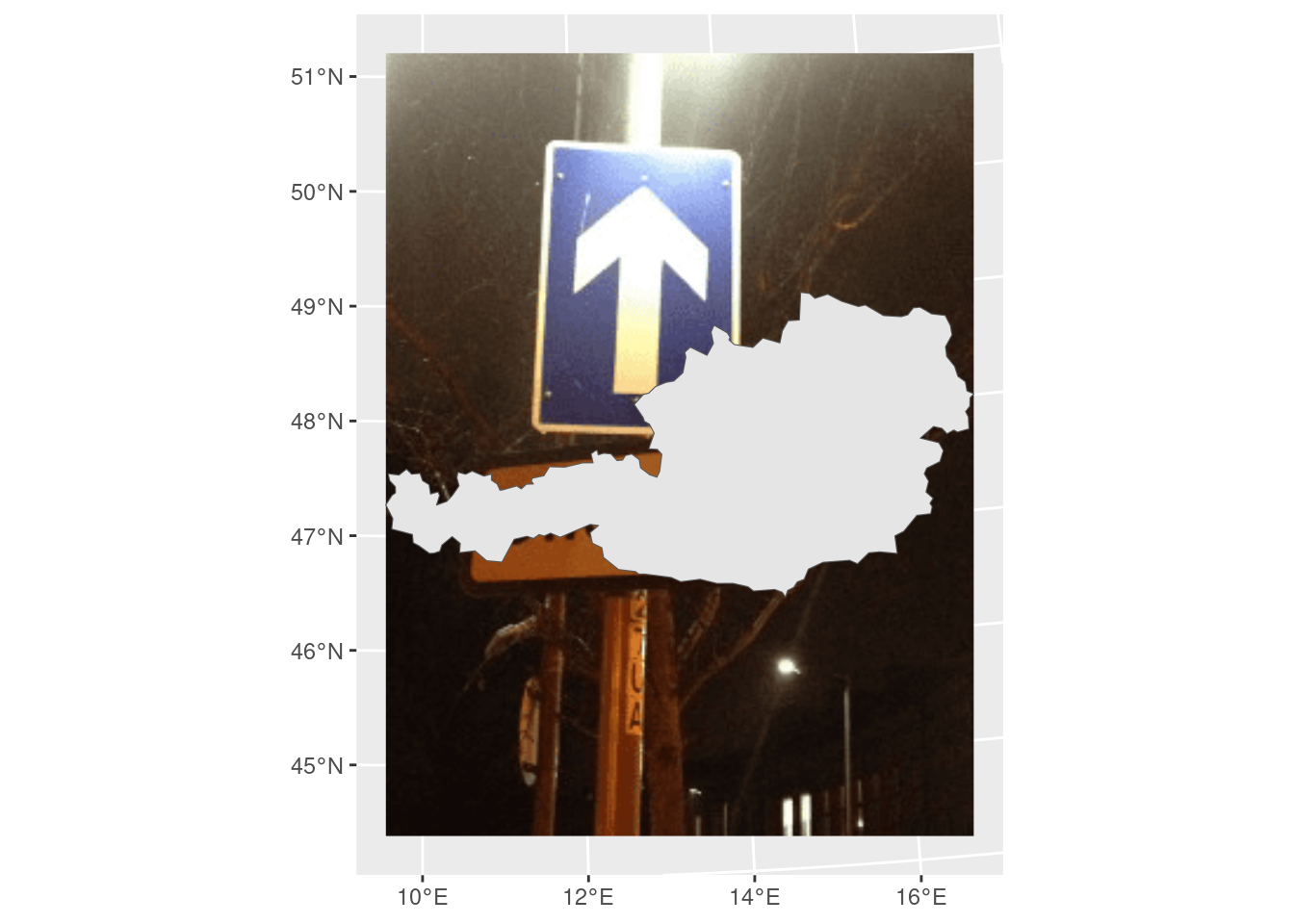# Get Started

Starting with `rasterpic` is very easy! You just need a image (`png`, `jpeg/jpg` or `tif/tiff`) and a spatial object (from the `sf` or the `terra`) package to start using it.

## Basic usage

We use here as an example the shape of Austria:

``````library(sf)
library(terra)
library(rasterpic)

# Plot
library(tidyterra)
library(ggplot2)

# Shape and image
x <- read_sf(system.file("gpkg/austria.gpkg", package = "rasterpic"))
img <- system.file("img/vertical.png", package = "rasterpic")

# Create!

default <- rasterpic_img(x, img)

autoplot(default) +
geom_sf(data = x)``````## Options

The function provides several options for expanding, alignment and cropping.

### Expand

With this option the image is zoomed out of the spatial object:

``````expand <- rasterpic_img(x, img, expand = 1)

autoplot(expand) +
geom_sf(data = x)``````